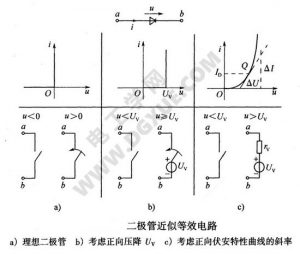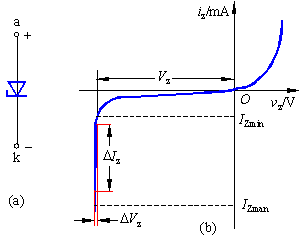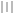# 半导体二极管

• 将PN结等装起来，从P区引出的电极为阳极，从N区引出的电极为阴极.

## 1.2.2二极管的伏安特性

### 二极管和PN结伏安特性曲线的区别

• 与PN结一样，二极管具有单向导电性.但是，由于二极管内存在半导体电阻和引线电阻，当外加正向电压，在相同的电流强度时，二极管的端电压大于PN结上的压降.也就是说：在外加正向电压相同的情况下，二极管的电流要小于PN结的电流.另外，由于二极管表面存在漏电流的情况，使得外加反向电压时的反向电流增大.
• 在近似分析时，二极管的电流仍然可以采用式$(1.1.2)、(1.1.3)$来描述二极管的伏安特性，即：
\begin{aligned} i = I_S(e^{\frac{qu}{kT}} - 1) \ = I_S(e^{\frac{u}{U_T}} - 1) \end{aligned}
• 实际测量时，只有在正向电压足够的大时，正向电流才会从零随端电压按指数规律增大.使二极管开始导通的电压称为开启电压$U_{on}$.### 温度对二极管伏安特性的影响

• 环境温度升高时，二极管的正向伏安特性曲线将会左移，反向伏安特性曲线将下移.

### 二极管的主要参数

• 为描述二极管的性能，常引用以下几个主要参数：
• 最大整流电流$I_F$
$I_F$是二极管长期运行时允许通过的最大正向平均电流，其值与PN结面积及外部散热条件等有关.
• 最大反向工作电压$U_R$
$U_R$是二极管工作时允许外加的最大反向电压通常$U_R$为击穿电压$U_{BR}$的一半.
• 反向电流$I_R$
$I_R$是二极管未击穿时的反向电流，$I_R$越小，二极管的单向导电性能越好，$I_R$对温度非常敏感.
• 最高工作频率$f_M$
$f_M$ 是二极管工作时的上限截止频率，前面我们曾说在高频率下需要考虑PN结的结电容$C_j$，也就是说，超过$f_M$ 时二极管将不能很好地体现单向导电性.

## 1.2.4二极管的等效电路

### 由伏安特性折线化得到的等效电路

• 图（a）所示的折线化伏安特性表明二极管导通时正向电压降为零，截 止时反向电流为零，称为理想二极管，用空心的二极管符号表示.
• 图（b）所示表明二极管正向导通时压降为一个常量$U_{on}$，截止时反向电流为零.因此等效电路是理想二极管串联电压源.
• 图（c）所示表明当二极管正向电压U大于$U_{on}$后其电流I与U成线性关系，直线斜率为$\frac{1}{r_D}$.二极管截止时反向电流为零.因此等效电路是理想二极管串联电压源和电阻.### 二极管的微变等效电路

• 将二极管等效为一个动态小电阻，利用二极管的电流方程可以得到：
\begin{aligned} \frac{1}{r_D} = \frac{\mathrm{di}}{\mathrm{du}} = \frac{\mathrm{d[I_S(e^{\frac{u}{U_T}} - 1)]}}{du} = \frac{I_S}{U_T} \cdot e^{\frac{u}{U_T}} ≈\frac{I_D}{U_T} \end{aligned}
即：
\begin{aligned} r_d ≈ \frac{U_T}{I_D}\end {aligned} \tag{1.2.1}
式中的$I_D$是二极管伏安特性曲线上的一点对应的电流.

## 1.2.5稳压二极管

• 稳压二极管在反向击穿时，在一定的电流范围内，端电压几乎保持不变，表现出稳压特性.

### 稳压管的伏安特性

• 稳压二极管的正向曲线为指数曲线，当稳压管被反向击穿时，击穿区的曲线很陡，几乎平行于纵轴，表现出稳压特性.### 稳压管的主要参数

• 稳定电压$U_Z$
$U_Z$是在规定电流下稳压管的反向击穿电压
• 稳定电流$I_Z$
$I_Z$是稳压管工作在稳压状态时的参考电流，电流低于这个值时稳压效果变坏，也是保障稳压特性的最小电流
• 额定功耗$P_{ZM}$
$P_{ZM}$等于稳压管的稳定电压与最大稳定电流的乘积.稳压管的功耗超过这个数值时，稳压管会损坏.
• 动态电阻$r_z$
$r_z$是稳压管工作在稳压区时，端电压的变化量与其电流变化量之比.
• 温度系数$\alpha$
$\alpha$表示温度没变化1℃时稳压值的变化量.即$\alpha = \Delta U_Z/\Delta T$.
• 稳定电压$U_Z$小于4V的管子具有负的温度系数，属于齐纳击穿，温度升高时稳定电压值下降
• 稳定电压$U_Z$大于7V的管子具有正的温度系数，属于雪崩击穿，温度升高时稳定电压值上升
• 稳定电压$U_Z$在4~7V之间的管子温度系数非常小，近似为零.
• 稳压管的电路中需要串联一个限流电阻来保障稳压管正常工作.Invitation
USTC-茶糜花开
FeynmanDirac
created:12/03/2022
Welcome to USTC-茶糜花开

This is an identification card as an honored membership of FeynmanDirac

Happy to see you follow FeynmanDirac, enjoy science together验证码启动中...